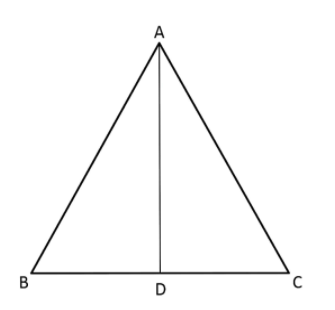QuestionAnswers

# In triangle $\vartriangle$ ABC, AD is perpendicular to BC and $A{D^2} = {\text{ }}BD \times DC$. Prove that angle $\angle BAC{\text{ }} = {\text{ }}90^\circ$.Hint: Apply Pythagoras theorem in right angled $\vartriangle ADC \& \vartriangle ABD$ and make equations of relation between different sides of the triangle in such a way that we can apply converse of Pythagoras theorem to prove $\angle BAC{\text{ }} = {\text{ }}90^\circ$

Complete step by step solution:
In right angle triangle $\vartriangle ABD$, $AD$ is perpendicular to $BC$, $AD \bot BC$
$\angle ABD = \angle ADC = 90^\circ$
Required to prove: $\angle BAC{\text{ }} = {\text{ }}90^\circ$
To prove, we will have to apply Pythagoras theorem in right angled triangle $\vartriangle ADC\& \vartriangle ABD$
Pythagoras' Theorem states that, for any right-angled triangle, the area of the square on the hypotenuse is equal to the sum of the areas of the squares on the two shorter sides.
By applying Pythagoras theorem in right angled $\vartriangle$ADB,
${\text{Hypotenuse}}^2 = {\text{Perpendicular}}^2 + {\text{Base}}^2$
$\Rightarrow A{B^2} = A{D^2} + B{D^2}$
$\Rightarrow A{D^2} = A{B^2} - B{D^2}$…………………. (1)
By applying Pythagoras theorem in right angled $\vartriangle$ADC,
$\;A{C^2} = A{D^2} + C{D^2}$
$\; \Rightarrow A{D^2} = A{C^2} - C{D^2}$………………… (2)
$\; \Rightarrow A{C^2} - C{D^2} + A{B^2} - B{D^2} = 2A{D^2}$$\Rightarrow B{D^2} + C{D^2} + 2\left( {BD \times DC} \right)$
Simplifying the above equation,
$\Rightarrow B{D^2} + C{D^2} + 2\left( {BD \times DC} \right) = A{B^2} + A{C^2}$
Arrange $BC{\text{ }}\& {\text{ }}DC$ terms in the same side
$\Rightarrow {\left( {BD + DC} \right)^2} = A{B^2} + A{C^2}$
By applying $\left[ {{\text{ }}BD{\text{ }} + {\text{ }}DC{\text{ }}={\text{ }}BC} \right]$formula,
$\Rightarrow {BC^2} = A{B^2} + A{C^2}$$\left[ {\because {\text{ }}BD{\text{ }} + {\text{ }}DC{\text{ }} = {\text{ }}BC} \right]$
By the converse of Pythagoras theorem,
If the square of the length of the longest side of a triangle is equal to the sum of the squares of the other two sides, then the triangle is a right triangle.
[As longest side of triangle ABC is $BC$ & other two sides are $AB$ and $AC$]

$\therefore \angle BAC{\text{ }} = {\text{ }}90^\circ$. Hence proved.

Note:
First of all, make a relatable diagram and then equations relating different sides of the triangle. We need to have concepts of converse of Pythagoras theorem to prove that $\angle BAC{\text{ }} = {\text{ }}90^\circ$
Right Angled Triangle ConstructionsCBSE Class 10 Maths Chapter 6 - Triangles FormulaCBSE Class 7 Maths Chapter 6 - Triangle and Its Properties FormulasCBSE Class 10 Maths Chapter 10 - Circles FormulaGroup Theory in MathematicsCBSE Class 10 Maths Chapter 8 - Introduction to Trigonometry FormulaCBSE Class 10 Maths Chapter 3 - Pair of Linear Equations in Two Variables FormulaCBSE Class 10 Maths Chapter 2 - Polynomials FormulaCBSE Class 10 Maths Chapter 14 - Statistics FormulaCBSE Class 10 Maths Chapter 15 - Probability FormulaImportant Questions for CBSE Class 10 Maths Chapter 6 - TrianglesImportant Questions for CBSE Class 10 Maths Chapter 10 - CirclesImportant Questions for CBSE Class 10 Maths Chapter 8 - Introduction to TrigonometryImportant Questions for CBSE Class 10 Maths Chapter 12 - Areas Related to CirclesImportant Questions for CBSE Class 10 Maths Chapter 3 - Pair of Linear Equations in Two VariablesImportant Questions for CBSE Class 7 Science Chapter 10 - Respiration in OrganismsImportant Questions for CBSE Class 10 Maths & Science with AnswersImportant Questions for CBSE Class 10 Maths Chapter 11 - ConstructionsImportant Questions for CBSE Class 10 Maths Chapter 15 - ProbabilityImportant Questions for CBSE Class 9 Maths Chapter 10 - CirclesCBSE Class 10 Maths Question Paper 2017CBSE Class 10 Maths Question Paper 2020Maths Question Paper for CBSE Class 10 - 2011Maths Question Paper for CBSE Class 10 - 2008Maths Question Paper for CBSE Class 10 - 2012Maths Question Paper for CBSE Class 10 (2016)Maths Question Paper for CBSE Class 10 - 2010Maths Question Paper for CBSE Class 10 - 2007Maths Question Paper for CBSE Class 10 - 2009CBSE Class 10 Maths Question Paper 2018 - Free PDFRS Aggarwal Class 9 Solutions Chapter-9 Congruence of Triangles and Inequalities in a TriangleNCERT Solutions for Class 10 Maths Chapter 6 TrianglesRS Aggarwal Class 10 Solutions - TrianglesRD Sharma Solutions for Class 9 Maths Chapter 10 - Congruent TrianglesRD Sharma Solutions for Class 10 Maths Chapter 4 - TrianglesNCERT Solutions for Class 10 Maths Chapter 6 Triangles in HindiNCERT Solutions for Class 7 Maths Chapter 6 The Triangle and Its Properties In HindiNCERT Solutions for Class 7 Maths Chapter 6 The Triangle and Its PropertiesNCERT Solutions for Class 10 Maths Chapter 10 Circles in HindiRD Sharma Solutions for Class 9 Maths Chapter 9 - Triangle and its Angles